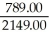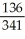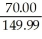# Quiz 6: Trade Discounts,cash Discounts,markup,and Markdown

Business

Amount of Discount = 2149 - 1360 = \$789.00 Rate of discount == 36.7148%

Amount of discount = 341 - 205 = \$136.00 Rate of discount == .39883 = 39.8827%

Amount of discount = 149.99-79.99 = 70.00 Rate of discount == .466697 = 46.670%